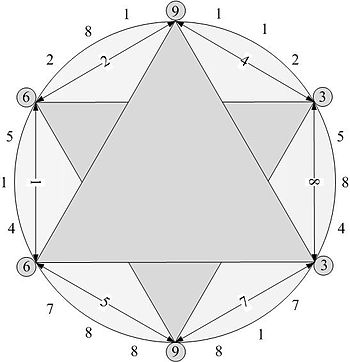# Fun with FunctionsEnglish: This image shows the 3-6-9 hexagram of the circle made of the digital root of Fibonacci numbers. (Photo credit: Wikipedia)

I'm building a language designed to be natural with linked data just as programs today are natural with local memory. The result is highly functional and data-relative in nature and reminds me of how XSLT works relative to XML nodes (e.g., a current node, child nodes, ancestor nodes). I have quite a few tests passing for the parser and core engine, so now I'm branching out into libraries and seeing what I can do with all of the pieces.

The first thing I want to do is define a stream of numbers starting with 1 and going up:

If you've done much functional programming before, you'll recognize the `upfrom_f(upfrom_f, ...)` pattern as the Y combinator. I have to do this because I can't use a symbol until after it's defined, and `upfrom_f` isn't defined until after the semicolon. The underscore is a placeholder indicating that the result is a curried function.

Now, I can use `upfrom(1)` (or `1..`) to give me a stream of integers starting at one and continuing for as many as I need. The engine will keep producing integers upon request.

## Add a Pinch of Selection

The next function I want to define lets me know if one integer is divisible by another:

The tilde inverts the truth (`0` being a false value and non-`0` being a true value for integers) of an expression or function. A number is divisible by another if the remainder is not non-zero.

We can now produce a stream of even numbers as easy as:

No sense starting at `1` since we know it will be odd. The percent sign is the functional equivalent of modulus: we're walking the stream accepting each element that fits with respect to the filtering function.

## Build out a Sieve

I have all the pieces to construct a Sieve of Eratosthenes. A stream of primes isn't very useful, especially any that result from the sieve in a reasonable running time, but this is a good example for demonstrating how a language thinks about data and algorithms.

The primes are just the list of numbers that don't get filtered out by the sieve.

The sieve allows the next number in the stream to be a prime (the `s'`), but then adds it on to the list of divisors that filter out subsequent numbers from the stream (`~divisible-by?(_, s') % s...`).

Another example is the Fibonacci sequence, which doesn't need a stream of numbers as a seed.

This produces the familiar sequence `1, 1, 2, 3, 5, 8, 13, 21, ...`.

## Mapping and Composition

I've used function inversion (`~`) and filtering (`%`), but there are two more tricks up my functional sleeve: mapping (`@`) and composition (`.`).

Let's start with a simple function that squares its argument:

Now, we can produce a sequence of squared primes or Fibonacci numbers:

If we only wanted the even squares for the Fibonacci numbers:

The only even square of a prime is `4`, so that's not much fun.

In this case, we could put the filter for `even?` and the `squared` mapping in either order since the square of an even number is even and the square of an odd number is odd. This isn't the case in general. For example, squaring after doubling and doubling after squaring will give different results: `squared @ doubled @ 1..` results in `[4, 16, 36, ...]`, but `doubled @ squared @ 1..` results in `[2, 8, 18, ...]`.

Some quick equivalences:

The curly braces (`{...}`) define an anonymous function, or lambda, for which the hash (`#`) is the single argument.

## Future Possibilities

I've used code that will run with the engine today up to this point. Now, I want to dive into some hypothetical things that I'm working towards. Keep in mind that I want to work with linked data, which means working within RDF patterns. Everything is subject to change.

This produces a Shared Canvas manifest with a filtered sequence of pages (canvases). Typical interactive environments such as Mathematica provide useful visualizations of results. In this case, my goal is to build an environment that would show you a paging interface to look through the manifest that results from running the above code.

A big open question is how to incorporate the equivalent of the JSON-LD context. In the Shared Canvas example, I assumed that I was working with the recommended context.